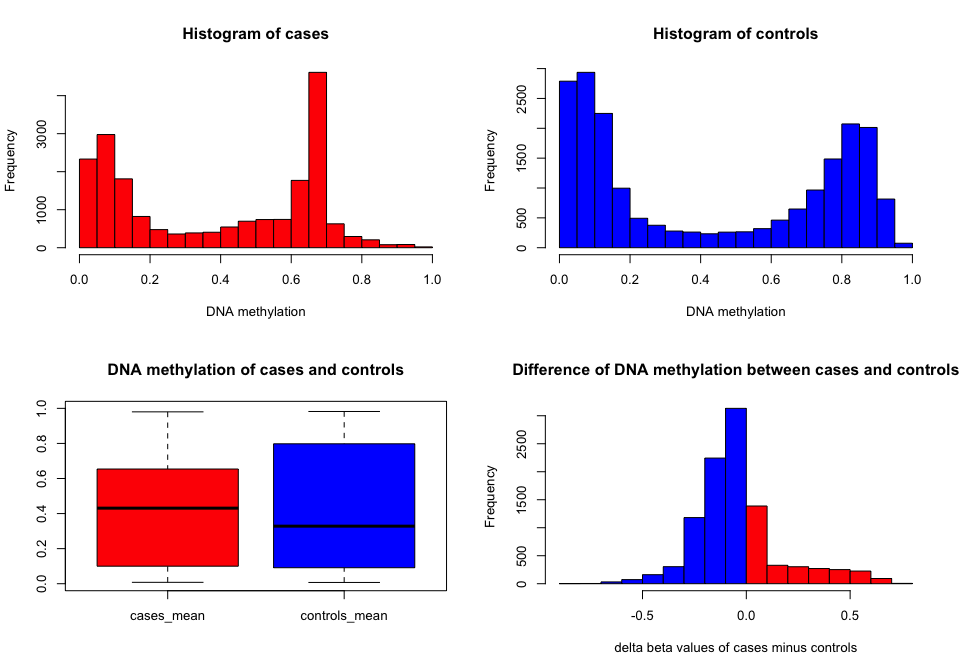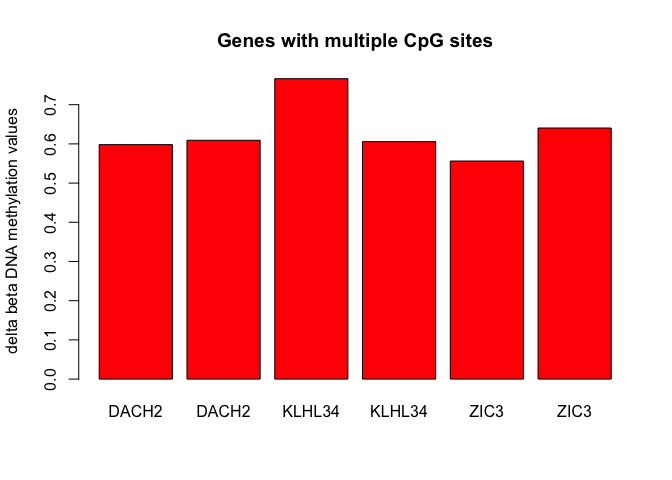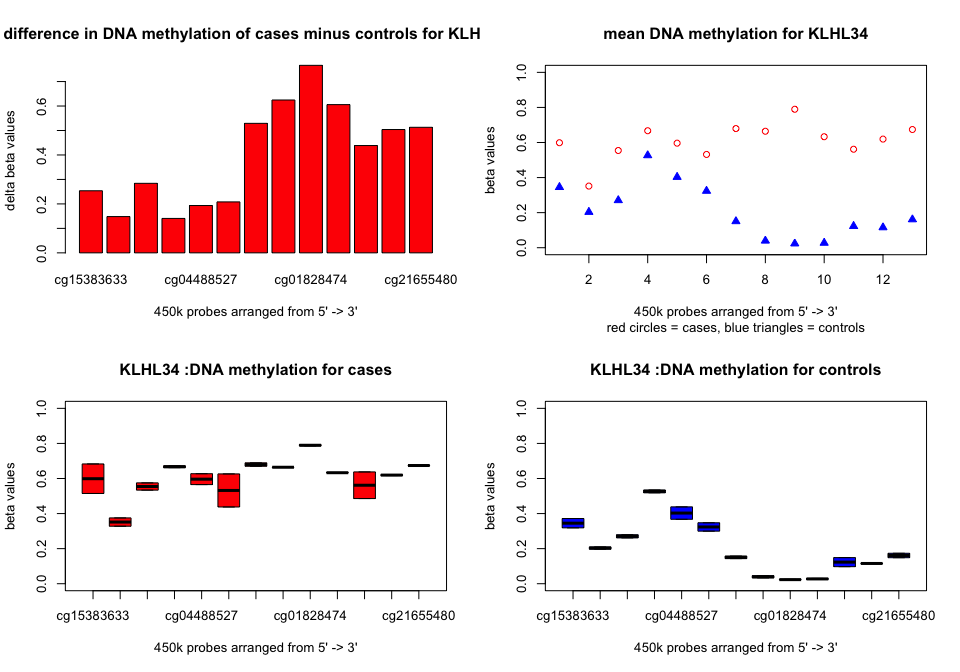# Array Based CpG Region Analysis Package (ABC.RAP)

#### 2016-10-20

ABC-RAP package was developed to analyse human 450k DNA methylation array data and to identify candidate genes that have significant differences in DNA methylation between cases and controls. The following example analysis is based on a small sample dataset “test_data” (included) containing 10,000 probes for 2 B-ALL cases and 2 controls from Busche et al (2013).

Busche S, Ge B, Vidal R, etc. Integration of high-resolution methylome and transcriptome analyses to dissect epigenomic changes in childhood acute lymphoblastic leukaemia. Cancer Research 2013; 73(14); 4323-4336.

library(ABC.RAP)
data("test_data")
data("nonspecific_probes")
data("annotation_file")

## Summary of the workflow

The package offers a choice of two workflows:

1. Step by step as follows

2. Using a single script (see “using one script” section)

Below is the package workflow using nine functions, and each step is dependent on the previous function.

Filtering the nonspecific probes:

test_data_filtered <- filter_data(test_data)

Annotation based on “UCSC platform”:

test_data_annotated <- annotate_data(test_data_filtered)

## Browsing the data

This function provides a general overview for the DNA methylation between cases and controls. It produces 4 plots: the upper 2 plots show DNA methylation (distribution) for cases (left) and controls (right). The left bottom plot compares the DNA methylation between cases and controls, and the right bottom plot represents the difference in DNA methylation between cases and controls (cases minus controls). Also, summary statistics for the difference in mean DNA methylation between cases and controls is produced.

Function arguments:

x = the filtered 450k probes from filter_data() function. In this example, it is “test_data_filtered”.

cases_column_1 = the first column (column number) for cases in the filtered dataset. In this example, it is column 1.

cases_column_n = the last column (column number) for cases in the filtered dataset. In this example, it is column 2.

controls_column_1 = the first column (column number) for controls in the filtered dataset. In this example, it is column 3.

controls_column_n = the last column (column number) for controls in the filtered dataset. In this example, it is column 4.

plot_data(test_data_filtered, 1, 2, 3, 4)##      Min.   1st Qu.    Median      Mean   3rd Qu.      Max.
## -0.881100 -0.171900 -0.038130 -0.041150  0.004206  0.774300

## Applying t-test

This function applies a “two.sided”, unequal variance t-test analysis, then selects p-values that are less than or equal to the cutoff value entered. For this example, a cutoff value of 1e-3 is used:

test_data_ttest <- ttest_data(test_data_filtered, 1, 2, 3, 4, 1e-3)

Checking number of rows from t-test output:

nrow(test_data_ttest)
##  156

## Delta beta analysis

This function calculates the difference between the beta values of cases and controls. It requires the minimum desired difference in proportion of DNA methylation for cases minus controls (delta_meth) and for controls minus cases (delta_unmeth). In this example, delta_meth is 0.5 and delta_unmeth is -0.5 which are based on the values from summary statistics from plot_data() function. Also it provides the option to specify probes where the average beta value of the cases or controls is greater than a cutoff value (e.g. 0.94) or less than a cutoff value (e.g. 0.06).

test_delta_beta <- delta_beta_data(test_data_filtered, 1, 2, 3, 4, 0.5, -0.5, 0.94, 0.06)

Checking the number of rows from delta beta analysis:

nrow(test_delta_beta)
##  187

## Overlapping t-test and delta beta outputs

The following function overlaps the results of the previous 2 analyses:

test_overlapped_data <- overlap_data(test_data_ttest, test_delta_beta)

Checking the number of rows (CpG sites) that are overlapping between the two analyses:

nrow(test_overlapped_data)
##  26

## Identifying genes for which multiple CpG sites show significant methylation differences:

test_CpG_hits <- CpG_hits(test_overlapped_data)

Gene names and their number of significantly different CpG sites:

test_CpG_hits
##         Var1 Freq
## 5160   DACH2    2
## 10191 KLHL34    2
## 22617   ZIC3    2

Plotting the candidate genes:

plot_candidate_genes(test_overlapped_data)## Investigating candidate genes:

“plot_gene” function generates four plots for any investigated gene: plot 1 (top left) shows the difference in beta values between cases and controls for each probe; plot 2 (top right) shows the mean methylation level for cases (red circles) and controls (blue triangles); and plots 3 and 4 (bottom plots) show the distribution of DNA methylation for each probe, for cases and controls, respectively. Also, an annotation table for all probes arranged from 5’ to 3’ is generated with the following columns: probe names, gene name, distance from transcription start site (TSS), mean methylation for cases, mean methylation for controls, delta beta (cases minus controls), and t-test p.value. KLHL34 is used as an example:

Function arguments:

x = the filtered and annotated 450k probes. In this example, it is “test_data_annotated”

b = gene name between quotation marks. In this example, “KLHL34” is used.

KLHL34 <- plot_gene(test_data_annotated, "KLHL34", 1, 2, 3, 4)##              Gene Chr      Distance Relation_to_CGI cases_mean
## cg15383633 KLHL34   X       1stExon          Island  0.5987974
## cg10607675 KLHL34   X       1stExon          Island  0.3515215
## cg01640808 KLHL34   X       1stExon          Island  0.5543636
## cg01563671 KLHL34   X       1stExon          Island  0.6671249
## cg04488527 KLHL34   X       1stExon          Island  0.5962242
## cg02812399 KLHL34   X 5'UTR;1stExon          Island  0.5318288
## cg14232291 KLHL34   X        TSS200          Island  0.6795550
## cg01891172 KLHL34   X        TSS200          Island  0.6641148
## cg01828474 KLHL34   X        TSS200          Island  0.7895418
## cg20312916 KLHL34   X        TSS200          Island  0.6329774
## cg12423482 KLHL34   X       TSS1500          Island  0.5615458
## cg06775759 KLHL34   X       TSS1500          Island  0.6194449
## cg21655480 KLHL34   X       TSS1500          Island  0.6740254
##            controls_mean delta_beta ttest_p.value
## cg15383633    0.34515336  0.2536440  0.1789289595
## cg10607675    0.20330812  0.1482134  0.0828907511
## cg01640808    0.27025886  0.2841047  0.0220891395
## cg01563671    0.52643590  0.1406890  0.0085029358
## cg04488527    0.40282047  0.1934037  0.0560646542
## cg02812399    0.32363093  0.2081978  0.2553849825
## cg14232291    0.15033859  0.5292164  0.0008604134
## cg01891172    0.03948140  0.6246334  0.0047339375
## cg01828474    0.02328138  0.7662605  0.0006825483
## cg20312916    0.02726248  0.6057149  0.0009448970
## cg12423482    0.12302873  0.4385171  0.0825854228
## cg06775759    0.11546027  0.5039846  0.0016310962
## cg21655480    0.16096001  0.5130654  0.0152313076

## Using one script:

Here is one script that applies all the previous scripts and produce plots for candidate genes automatically. The function exports two files onto the current working directory: 1. “process.ABC.RAP.plots.pdf” containing plots for all the candidate genes, and 2. “process.ABC.RAP.tables.txt” containing the annotation tables for the candidate genes.

Function arguments on the following order:

x = The normalised beta values in a data matrix format, where conditions are arranged in columns and cg probes are arranged in rows. In this example, it is “test_data”.

cases_column_1 = the first column (column number) for cases in the filtered dataset. In this example, it is column 1.

cases_column_n = the last column (column number) for cases in the filtered dataset. In this example, it is column 2.

controls_column_1 = the first column (column number) for controls in the filtered dataset. In this example, it is column 3.

controls_column_n = the last column (column number) for controls in the filtered dataset. In this example, it is column 4.

ttest_cutoff = the cutoff level to filter insignificant p-values. In this example, a cutoff value of 1e-3 is used.

meth_cutoff = the cutoff level for the methylation difference between cases and controls (cases minus controls). In this example, a cutoff value of 0.5 is used.

unmeth_cutoff = the cutoff level for the methylation difference between controls and cases (cases minus controls), consequently it is a negative value. In this example, a cutoff value of -0.5 is used.

high_meth = the upper margin for the desired highly methylated probes. In this example, a value of 0.94 is used.

low_meth = the lower margin for the desired highly unmethylated probes. In this example, a value of 0.06 is used.

process.ABC.RAP(test_data, 1, 2, 3, 4, 1e-3, 0.5, -0.5, 0.94, 0.06)